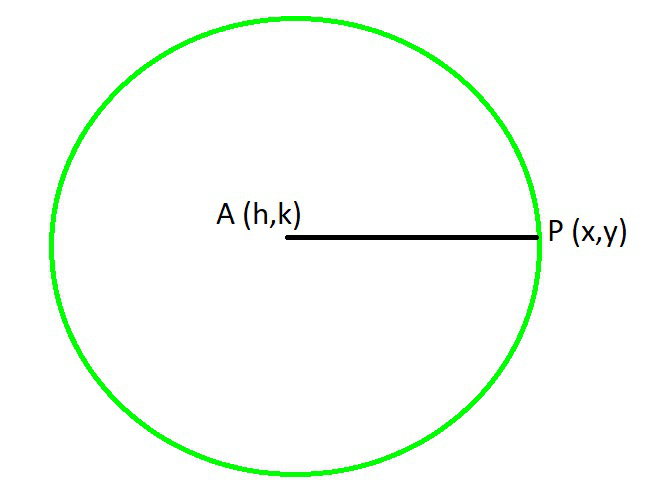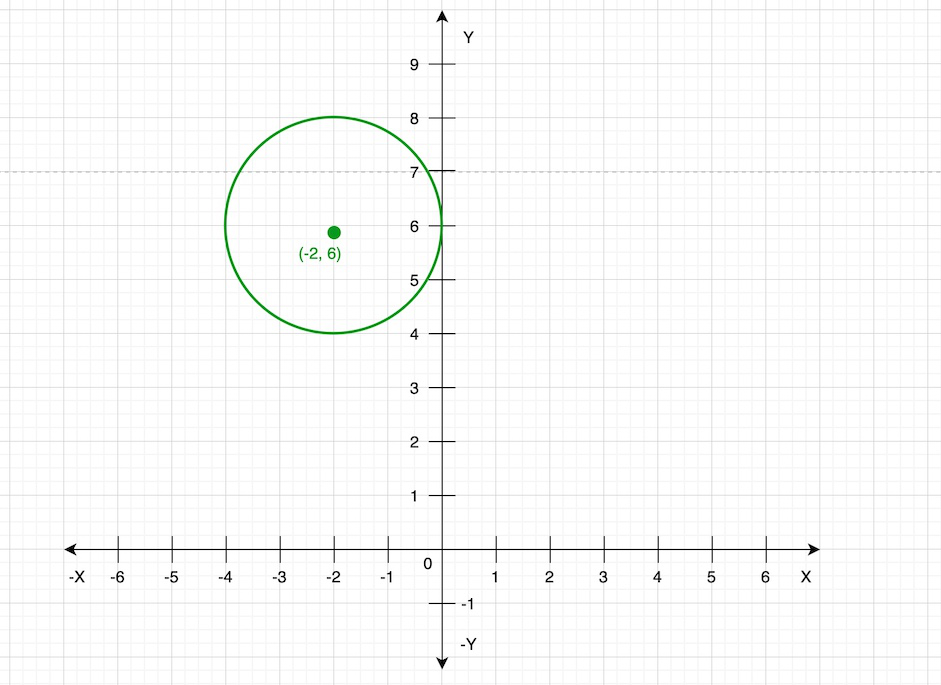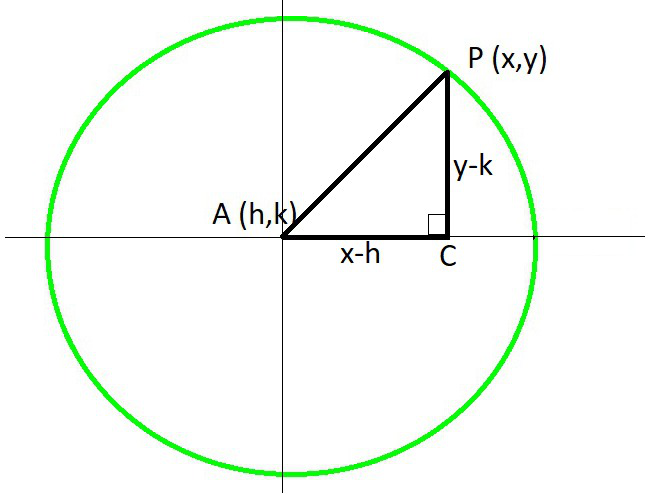# Equation of a Circle

• Difficulty Level : Hard
• Last Updated : 10 May, 2021

In mathematics, a circle is a shape that contains all the points in the plane and these points are equidistant from the given point. This given point is known as the centre of the circle. Or in other words, it is a curve that is traced by a point that moves in a plane such that its distance from a fixed point in that plane is always constant. The distance between a point on the circle to the centre of the circle is known as the radius of the circle and when a chord passes through the centre of the circle is known as diameter or the circle.

### Standard equation of a circle

To find the standard from of the circle let us assume that A(h, k) be the centre of the circle and r be the radius of the circle and P(x, y) be any point on the circumference.Then, AP = r …..(i)

Now using the distance formula we find the value of AP

AP =Now put the value of AP in eq(i), we get= r

Or squaring both side we get

(x – h)2 + (y – k)2 = r

This is the relation between the coordinates of any point on the circumference and hence it is the required equation of the circle having centre at A(h, k) and radius equal to r. Now we will see the variation in the Standard equation of a circle:

Case 1: When centre of the circle is at the origin(0, 0) and radius in r.
h = 0 and k = 0
On substituting in the standard equation of circle, we get
x2 + y2 = r2

Case 2: When the circle passes through the origin.
Here, let centre be A(h, k) and O be the origin which passes through circle.
Draw AM⊥OM
In △AMO, OA2 = OM2 + AM2
r2 = h2 + k2
On substituting in the standard equation of circle, we get
(x – h)2 + (y – k)2 = h2 + k2
x2 + y2 – 2hx – 2ky = 0

Case 3: When circle touched x-axis
Here, let centre be A(h, k). Since the circle touches the x-axis,
k = r
On substituting in the standard equation of circle, we get
(x – h)2 + (y – r)2 = r2
x2 + y2 – 2hx – 2ry + h2 = 0

Case 4: When circle touched x-axis
Here, let centre be A(h, k). Since the circle touches the x-axis,
h = r
On substituting in the standard equation of circle, we get
(x – r)2 + (y – k)2 = r2
x2 + y2 – 2rx – 2ky + k2 = 0

Case 5: When the circle touched both the axes.
Here, h = k = r
On substituting in the standard equation of circle, we get
(x – r)2 + (y – r)2 = r2
x2 + y2 – 2rx – 2ry + r2 = 0

Case 6: When the circle passes through the origin and centre lies on x-axis.
Here, k = 0 and h = r
On substituting in the standard equation of circle, we get
(x – r)2 + (y – 0)2 = r2
x2 + y2 – 2rx = 0

Case 7: When the circle passes through the origin and centre lies on y-axis.
Here, h = 0 and k = r
On substituting in the standard equation of circle, we get
(x – 0)2 + (y – r)2 = r2
x2 + y2 – 2ry = 0

### Sample Problems

Question 1. Find centre and radius of the circle from the given equation:

x2 + (y + 2)2 = 9

Solution:

After rearranging, we get

(x – 0)2 + (y – (-2))2 = 32

On comparing with the standard equation of circle, we have

h = 0, k = -2 and r = 3

So the centre of the circle is (0, -2)

and the radius of the circle = 3

Question 2. Find centre and radius of the circle from the given equation:

x2 + y2 + 6x – 4y + 4 = 0

Solution:

We have,

(x2 + 6x) + (y2 – 4y) = – 4

To make it a perfect square identity, add and subtract 9 and 4,

(x2 + 6x + 9) + (y2 – 4y + 4) – 9 – 4 = – 4

(x + 3)2 + (y – 2)2 = – 4 + 4 + 9

(x – (-3))2 + (y – 2)2 = 9

(x – (-3))2 + (y – 2)2 =32

On comparing with the standard equation of circle, we have

h = -3, k = 2 and r = 3

So the centre of the circle is (-3, 2)

and the radius of the circle = 3

### Graphing a circle from its standard equation

As we know that the standard equation of the circle is

(x – h)2 + (y – k)2 = r

Here, the centre of the circle is (h, k) and the radius of the circle is r. Now let us take an example:

The equation of the circle is (x + 2)2 + (y – 6)2 = 4

we can rewrite this equation as

(x – (-2))2 + (y – 6)2 = 22

On comparing with the standard equation of the circle we get

The centre of the circle is (-2, 6) and the radius of the circle is 2.

Now we will draw a circle on the graph.

Step 1: Draw x and y-axis

Step 2: Plot the centre of the circle on the graph that is (-2, 6)

Step 3: Mark any four points in the four directions from the centre of the circles and the distance between the points and the centre is 2.

Step 4: Join all these points to get a circle.### Expanded equation of a Circle

Now we will find the expanded equation of a circle. So, let us assume that A(h, k) be the centre of the circle and r be the radius of the circle and P(x, y) be any point on the circumference.Then AP = r  …(i)

In right-angled △ACP,

AP2 = AC2 + PC2

r2 = (x – h)2 + (y – k)2

Expanding further, we get

(x – h)2 + (y – k)2 = r2

(x2 – 2hx + h2) + (y2 – 2ky + k2) = r2

and if we bring r2 to the left-hand side and rearrange we get

The expanded equation of circle:

x2 – 2hx + h2 + y2 – 2ky + k2 – r2 = 0

Example: Find centre and radius of the circle using the following equation: x2 + y2 – 4x + 6y = 12.

Solution:

Given: x2 + y2 – 4x + 6y = 12

We can write as

(x2 – 4x) + (y2 – 6y) = 12

By manipulating identity, we get

(x2 – 4x + 4) + (y2 6y + 9) – 9 – 4 = 12

(x2 – 4x + 4) + (y2 – 6y + 9) = 12 + 9 + 4

(x – 2)2 + (y – 3)2 = 25

(x – 2)2 + (y – 3)2 = 52

On comparing with the standard equation of circle, we have

h = 2, k = 3 and r = 5

### General Equation of a Circle

The general equation of a circle is:

x2 + y2 + 2gx + 2fy + c = 0

For all values of g, f and c.

Now, on adding g2 + f2 on both sides of the equation, we get

(x2 + 2gx + g2)+ (y2 + 2fy + f2) = g2 + f2 − c

As, (x + g)2 = x2+ 2gx + g2 and (y + f)2 =y2 + 2fy + f

(x + g)2 + (y + f)2 = g2 + f2 – c

(x – (-g))2 + (y – (-f))2 = g2 + f2 – c ……….(1)

On comparing eq(1) with the standard equation, we get

h = −g, k = −f

r2 = g2+ f2−c

where (h, k) is the centre and ‘r’ is the radius of the circle.

Hence,

x2 + y2 + 2gx + 2fy + c = 0, represents the circle with centre (−g, −f) and radius (r) equal to r2 = g2 + f2− c.

• If g2 + f2 > c, then the radius of the circle is real.
• If g2 + f2 = c, then the radius of the circle is zero which tells us that the circle is a point which coincides with the centre. Such type of circle is called a point circle
• g2 + f2 <c, then the radius of the circle become imaginary. Therefore, it is a circle having a real centre and imaginary radius.

Some features of the general equation x2 + y2 + 2gx + 2fy + c = 0 of the circle are as follows:

• It is quadratic in both x and y.
• Coefficient of x2 = y2. (It is advisable to keep the coefficient of x2 and y2 unity)
• There is no term containing xy i.e., the coefficient of xy is zero.
• It contains three arbitrary constants viz. g, f and c.

### Sample Problems

Question 1. Find the equation of a circle whose centre is (-3, -2) and radius 6.

Solution:

Given: h = -3, k = -2, and, r = 6

Using the standard equation of circle,

(x – h)2 + (y – k)2 = r

(x – (-3))2 + (y – (-2))2 = 62

(x + 3)2 + (y + 2)2 = 62

x2 + 6x + 9 + y2 + 4y + 4 = 36

Hence, the required equation is

x2 + y2 + 6x + 4y – 23 = 0

Question 2. Find the equation of a circle whose radius is 7 and the centre is at the origin.

Solution:

Given : r = 7 and the centre = (0, 0)

Using the standard equation of circle,

(x – h)2 + (y – k)2 = r

x2 + y2 = r2

x2 + y2 = 72

Hence, the required equation is

x2 + y2 – 49 = 0

Question 3. Find the radius and centre of the circle given by the equation

2x2 + 2y2 + 8x + 12y – 38  = 0

Solution:

In the given equation the coefficients of x2 and y2 are not unity.

Firstly, lets make them unity by dividing equation by 2,

x2 + y2 + 4x + 6y – 19 = 0

So, the coordinates of the centre are (-2, -3)

and, Radius =Radius =Question 4. Find the equation of the circle that passed through the points (1, 0), (-1, 0), and (0, 1).

Solution:

Let the required circle be

x2 + y2 + 2gx + 2fy + c = 0

As, it passes through (1, 0), (-1, 0), and (0, 1). Hence, they will satisfy the equation

By substituting them, we get

1 + 2g + c = 0 ……..(1)

1 – 2g + c = 0 ……..(2)

1 + 2f + c = 0 ……..(3)

From eq(1) and (2), we get

g = 0 and c = -1

Now, putting c = -1 in eq(3), we get

f = 0

Now, substituting the values of g, f and c in the main equation, we get

x2 + y2 = 1

Question 5. Find the equation of the image of the circle x2 + y2 + 16x – 8y + 64 = 0 in the line mirror x = 0.

Solution:

Equation given is:

x2 + y2 + 16x – 8y = – 64

(x2 + 16x) + (y2 – 8y) = – 64

On adding and subtracting 64 and 16, we get

(x2 + 16x + 64) + (y2 – 8y + 16) – 64 – 16 = – 64

(x2 + 16x + 64) + (y2 – 8y + 16) = – 64 + 64 + 16

(x + 8)2 + (y – 4)2 = 16

(x – (-8))2 + (y – 4)2 = 42

As, the radius of this circle is (-8, 4) and radius = 4.

The image of the circle in the line mirror will have its centre as (8, 4) and radius 4.

So, the equation will be,

(x – 8)2 + (y – 4)2 = 42

x2 + y2 – 16x – 8y + 64 = 0

My Personal Notes arrow_drop_up
Recommended Articles
Page :